• 软件应用大都选用图表设计来可视化各类软件数据，成功简化界面...然而，web\App设计之中的图表种类繁多，例如常见的饼形图、条形图、折线图、时刻表、示意图、流程图、直方图、散点图、点图等等，作为设计师和开发人
软件应用大都选用图表设计来可视化各类软件数据，成功简化界面设计的同时，也大幅提升其用户体验。此类图表设计, 为设计师们提供了更多随心创造和自我表达的空间， 其联动动效和交互设计，也为设计师们提供了更多界面提升和优化的可能性，设计师们也确实钟爱于在其实际的设计中，添加各类图表设计。

这也就解释了为什么如今图表会广泛出现在各类软件（包括Web和App）设计之中。

然而，web\App设计之中的图表种类繁多，例如常见的饼形图、条形图、折线图、时刻表、示意图、流程图、直方图、散点图、点图等等，作为设计师和开发人员，究竟如何才能完美设计和还原此类图表设计呢？不用担心！专注于UI资源素材的搜UI( SOOUI)，为大家带了app可视化图表设计模板一定会找到想要的答案。数据可视化接口设计数据可视化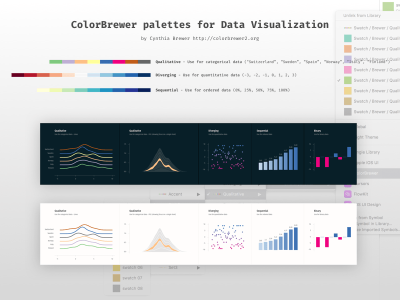调色板在ColorBrewer数据可视化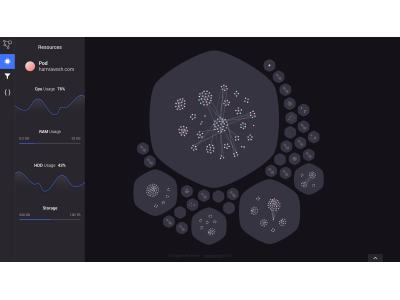Kubervis数据可视化

展开全文内置示例数据集 seaborn内置了十几个示例数据集，通过load_dataset函数可以调用。其中包括常见的泰坦尼克、鸢尾花等经典数据集。# 查看数据集种类
import seaborn as sns
sns.get_dataset_names()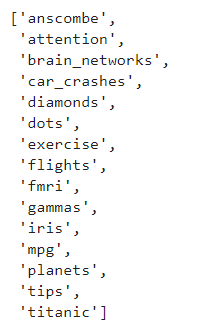import seaborn as sns
# 导出鸢尾花数据集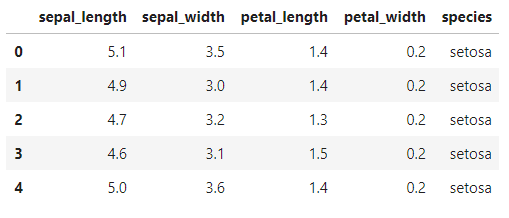1、散点图 函数sns.scatterplotimport seaborn as sns
sns.set()
import matplotlib.pyplot as plt
%matplotlib inline
# 小费数据集
ax = sns.scatterplot(x='total_bill',y='tip',data=tips)
plt.show()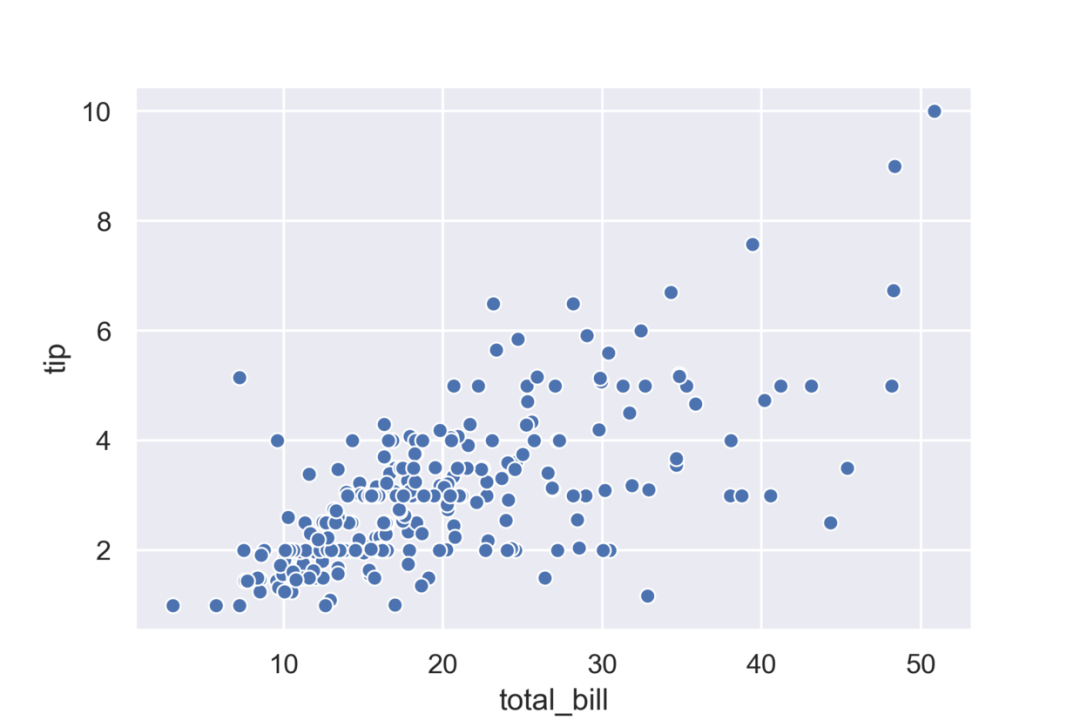2、条形图 函数sns.barplot显示数据平均值和置信区间import seaborn as sns
sns.set()
import matplotlib.pyplot as plt
%matplotlib inline
# 小费数据集
ax = sns.barplot(x="day", y="total_bill", data=tips)
plt.show()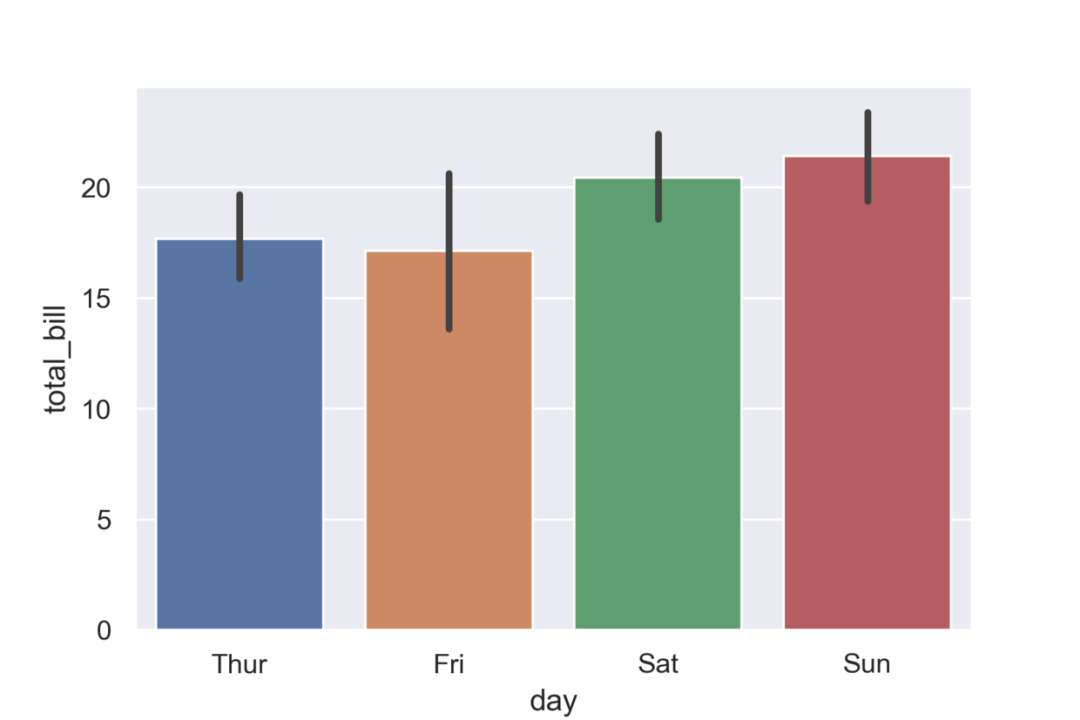3、线型图 函数sns.lineplot绘制折线图和置信区间import seaborn as sns
sns.set()
import matplotlib.pyplot as plt
%matplotlib inline
ax = sns.lineplot(x="timepoint", y="signal", data=fmri)
plt.show()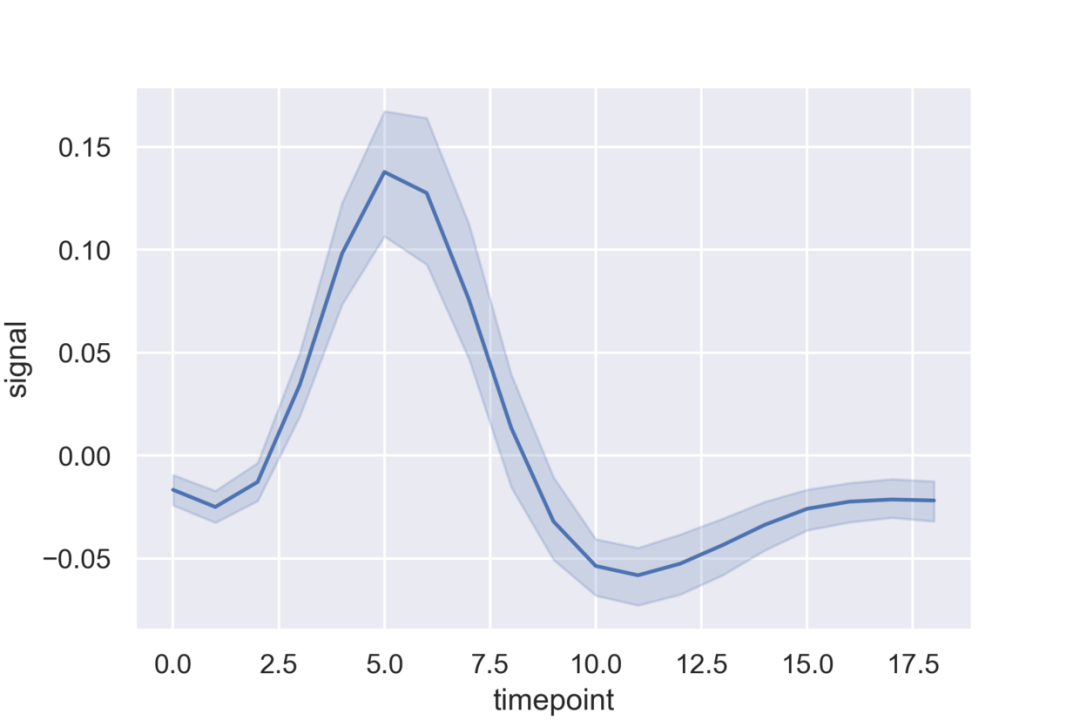4、箱线图 函数seaborn.boxplotimport seaborn as sns
sns.set()
import matplotlib.pyplot as plt
%matplotlib inline
ax = sns.boxplot(x="day", y="total_bill", data=tips)
plt.show()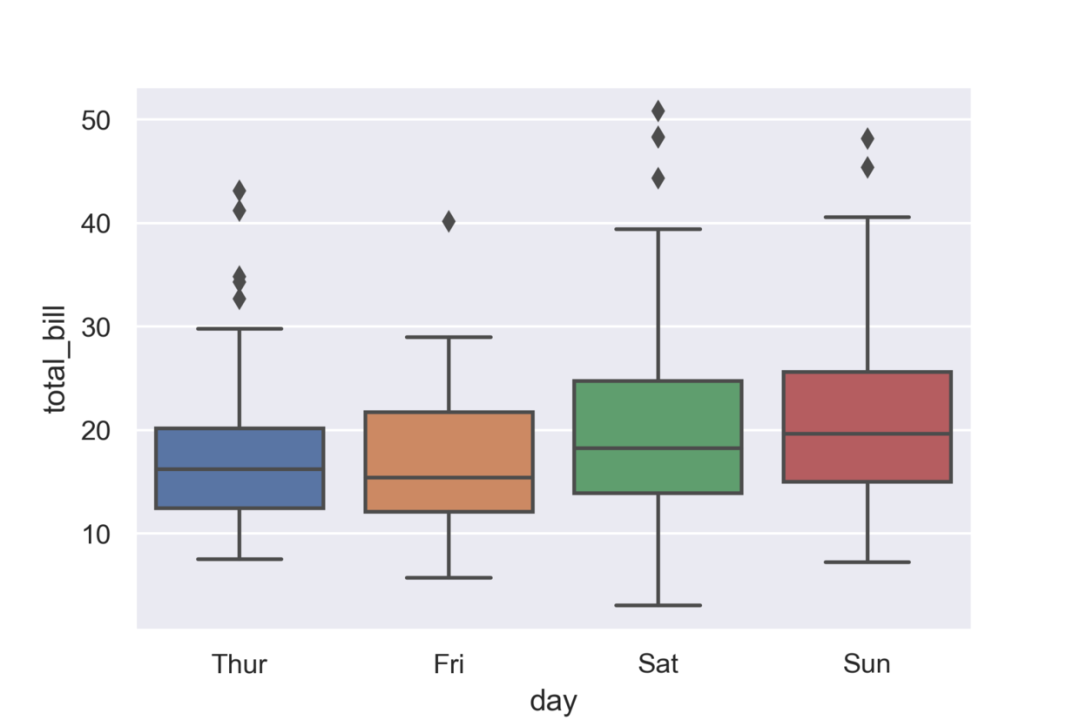5、直方图 函数seaborn.distplotimport seaborn as sns
import numpy as np
sns.set()
import matplotlib.pyplot as plt
%matplotlib inline
np.random.seed(0)
x = np.random.randn(1000)
ax = sns.distplot(x)
plt.show()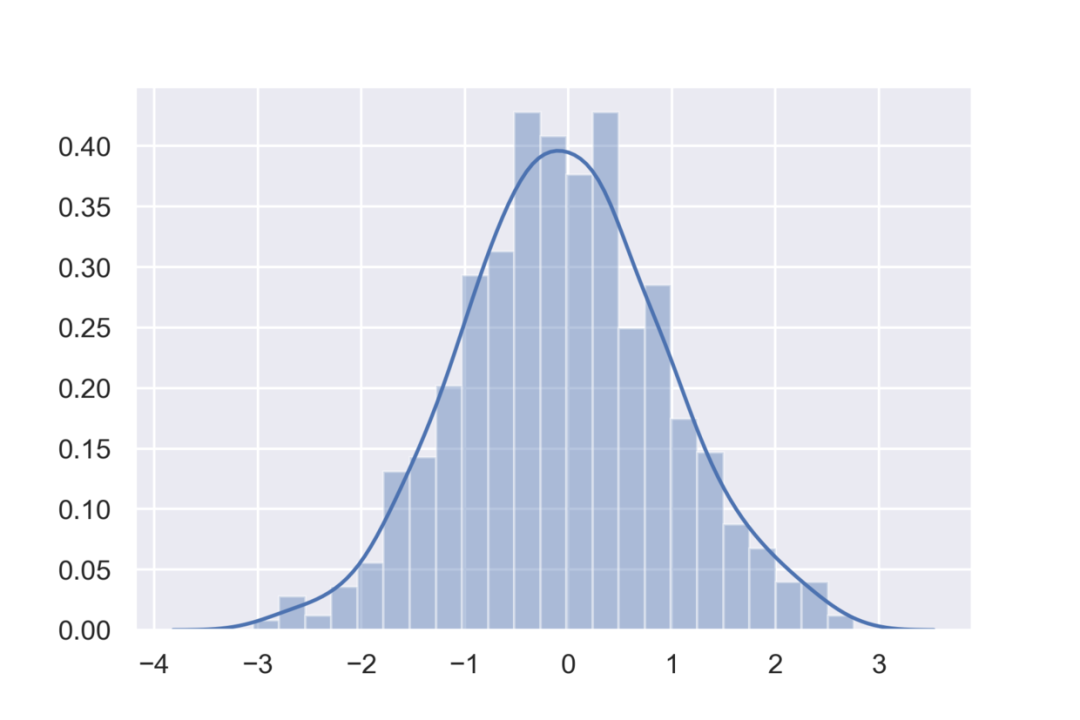6、热力图 函数seaborn.heatmapimport numpy as np
np.random.seed(0)
import seaborn as sns
sns.set()
import matplotlib.pyplot as plt
%matplotlib inline
uniform_data = np.random.rand(10, 12)
ax = sns.heatmap(uniform_data)
plt.show()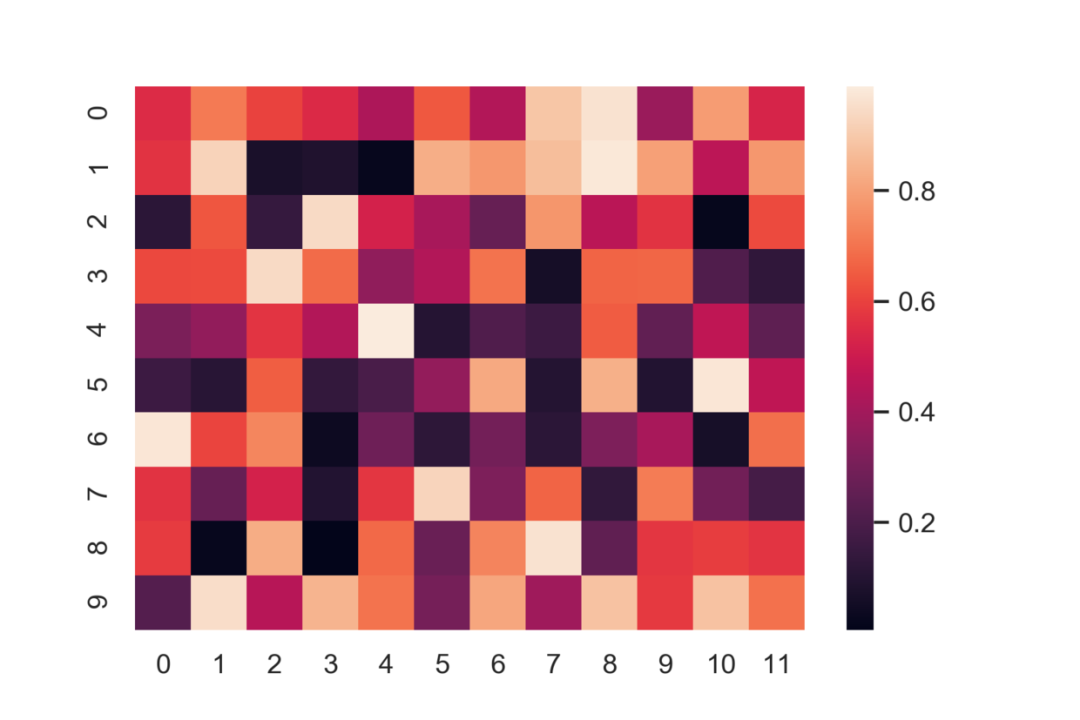7、散点图矩阵 函数sns.pairplotimport seaborn as sns
sns.set()
import matplotlib.pyplot as plt
%matplotlib inline
ax = sns.pairplot(iris)
plt.show()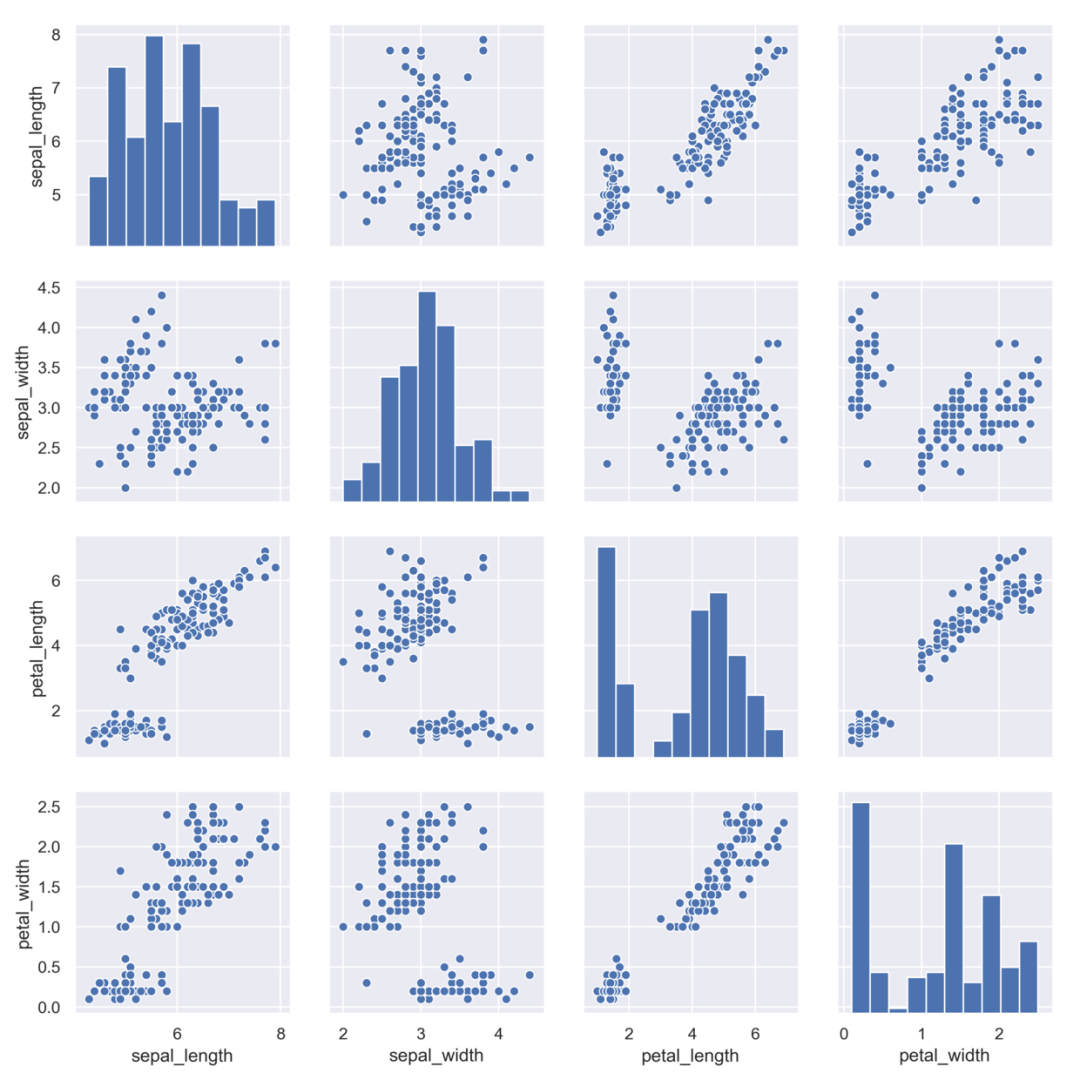8、分类散点图 函数seaborn.catplotimport seaborn as sns
sns.set()
import matplotlib.pyplot as plt
%matplotlib inline
ax = sns.catplot(x="time", y="pulse", hue="kind", data=exercise)\
plt.show()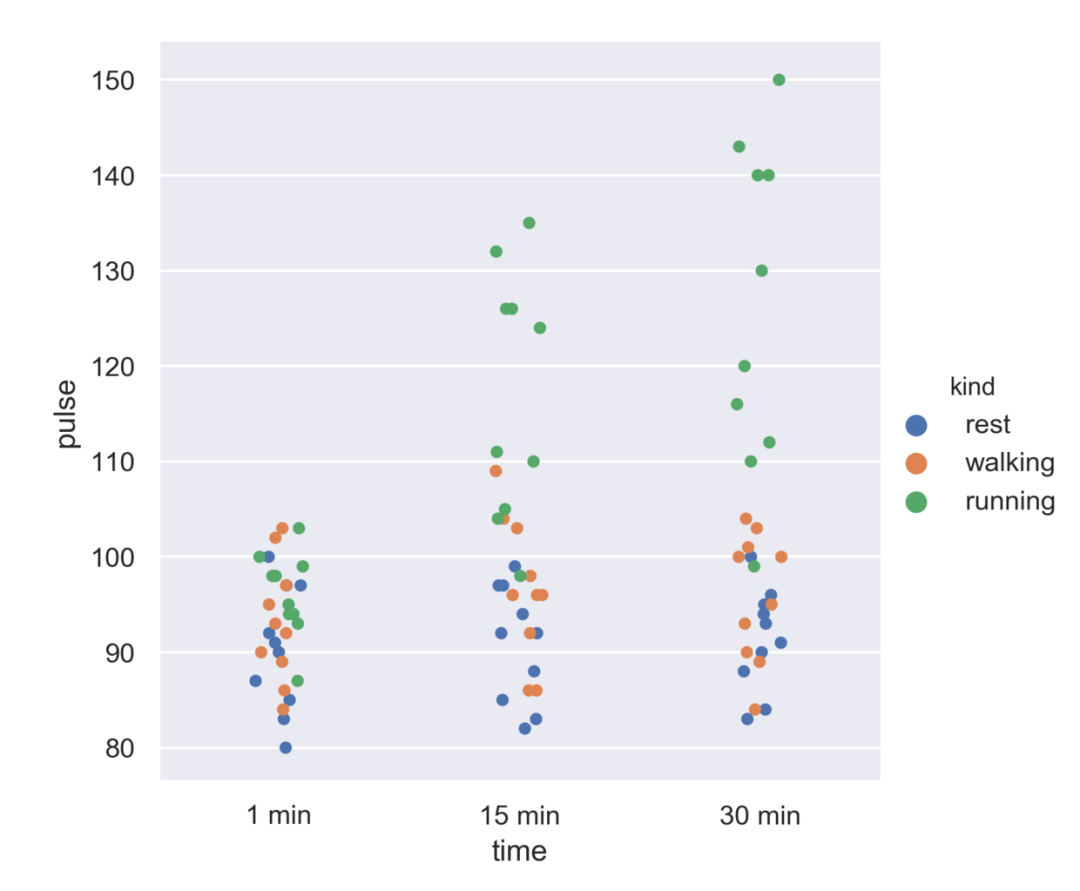9、计数条形图 函数seaborn.countplotimport seaborn as sns
sns.set()
import matplotlib.pyplot as plt
%matplotlib inline
ax = sns.countplot(x="class", data=titanic)
plt.show()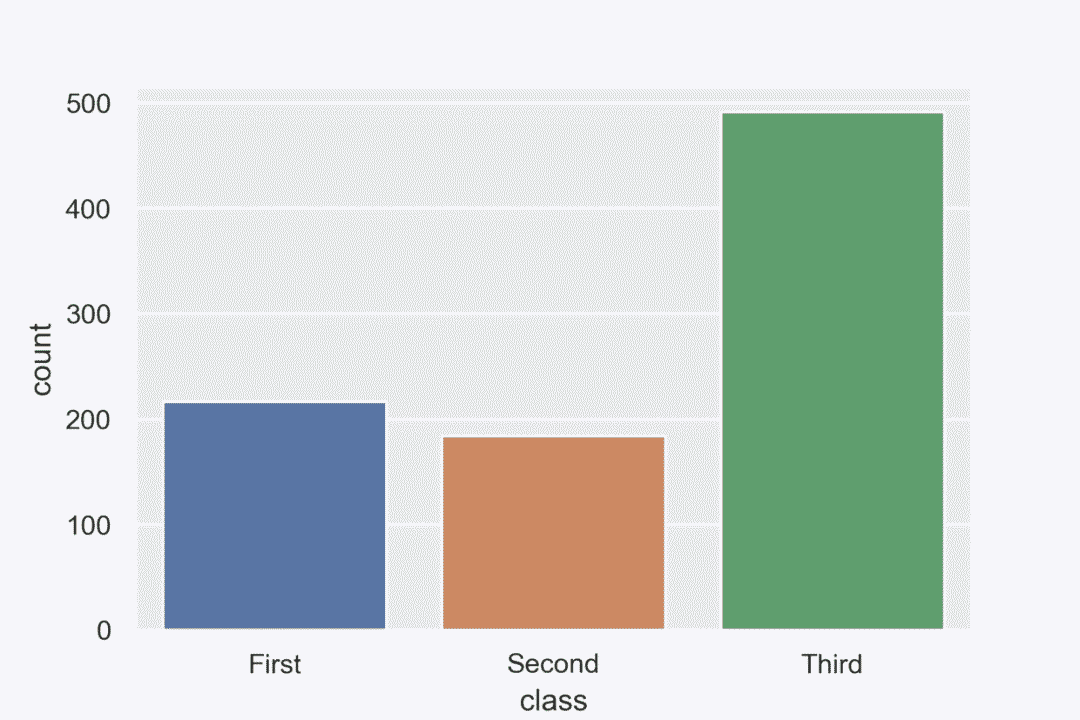10、回归图 函数 seaborn.lmplot绘制散点及回归图import seaborn as sns
sns.set()
import matplotlib.pyplot as plt
%matplotlib inline
ax = sns.lmplot(x="total_bill", y="tip", data=tips)

plt.show()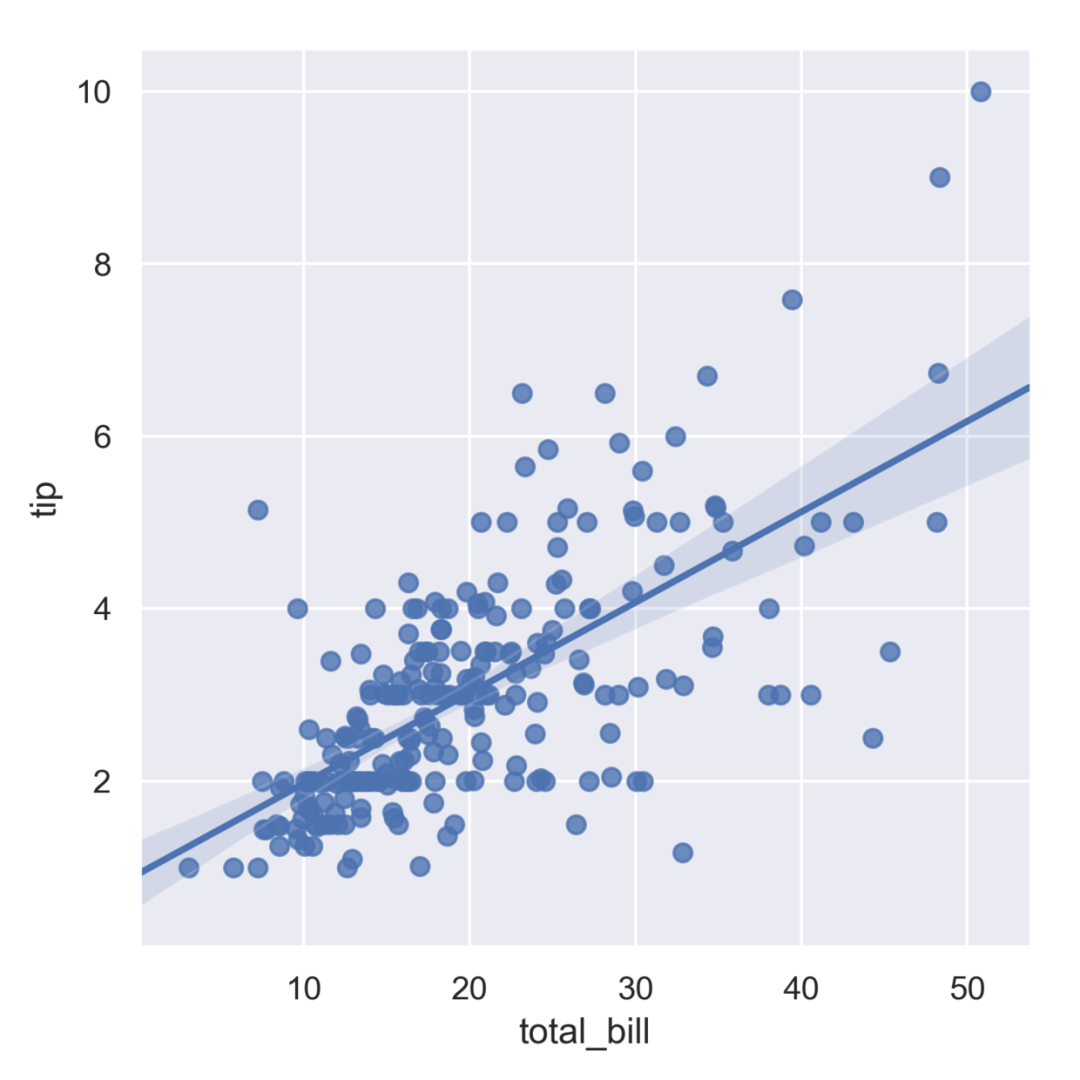精 彩 文 章 字节跳动只剩下小米这一个朋友了WebStorm超好用的10款插件，效率提升了好多！一文看懂：网址，URL，域名，IP地址，DNS，域名解析END来和小伙伴们一起向上生长呀~~~扫描下方二维码，添加小詹微信，可领取千元大礼包并申请加入 Python学习交流群，群内仅供学术交流，日常互动，如果是想发推文、广告、砍价小程序的敬请绕道！一定记得备注「交流学习」，我会尽快通过好友申请哦！（添加人数较多，请耐心等待）（扫码回复 1024  即可领取IT资料包）

展开全文• 其中包括常见的泰坦尼克、鸢尾花等经典数据集。# 查看数据集种类 import seaborn as sns sns.get_dataset_names() import seaborn as sns # 导出鸢尾花数据集 data = sns.load_dataset('iris') data.head...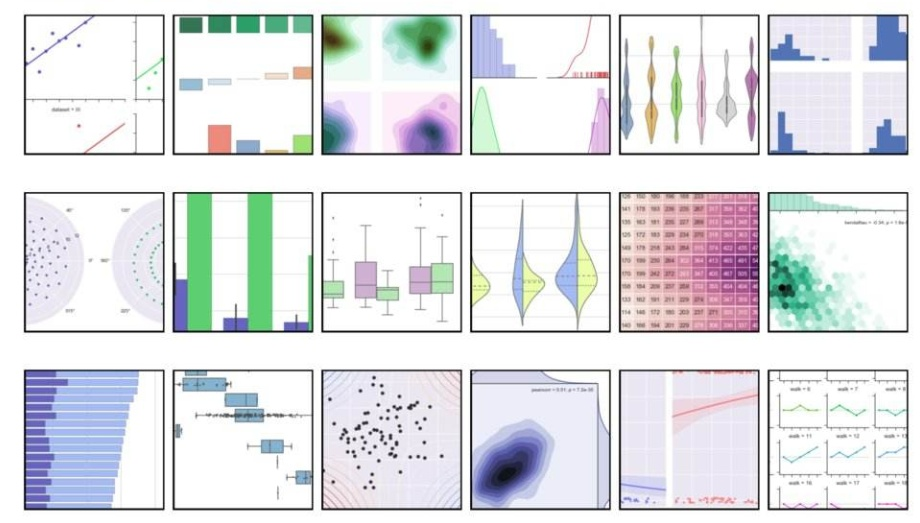内置示例数据集 seaborn内置了十几个示例数据集，通过load_dataset函数可以调用。其中包括常见的泰坦尼克、鸢尾花等经典数据集。# 查看数据集种类
import seaborn as sns
sns.get_dataset_names()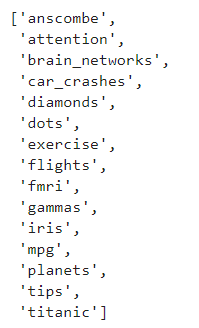import seaborn as sns
# 导出鸢尾花数据集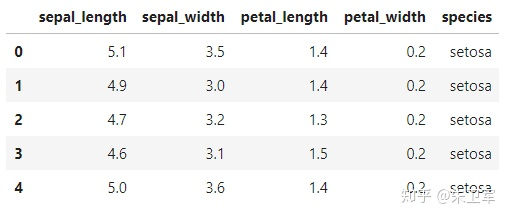1、散点图 函数sns.scatterplotimport seaborn as sns
sns.set()
import matplotlib.pyplot as plt
%matplotlib inline
# 小费数据集
ax = sns.scatterplot(x='total_bill',y='tip',data=tips)
plt.show()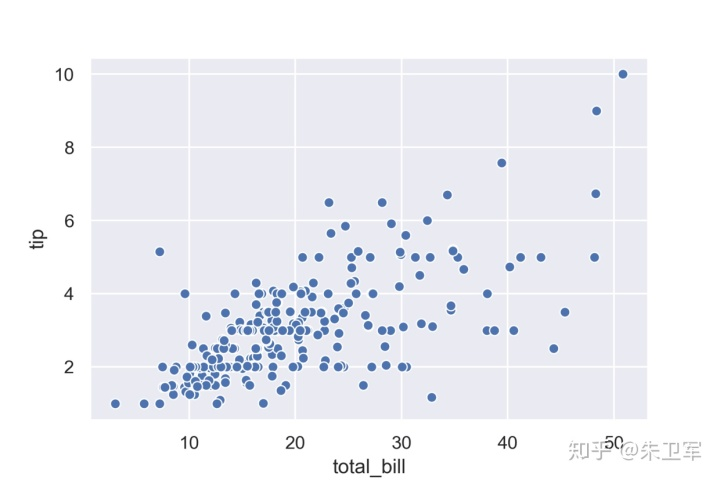2、条形图 函数sns.barplot显示数据平均值和置信区间import seaborn as sns
sns.set()
import matplotlib.pyplot as plt
%matplotlib inline
# 小费数据集
ax = sns.barplot(x="day", y="total_bill", data=tips)
plt.show()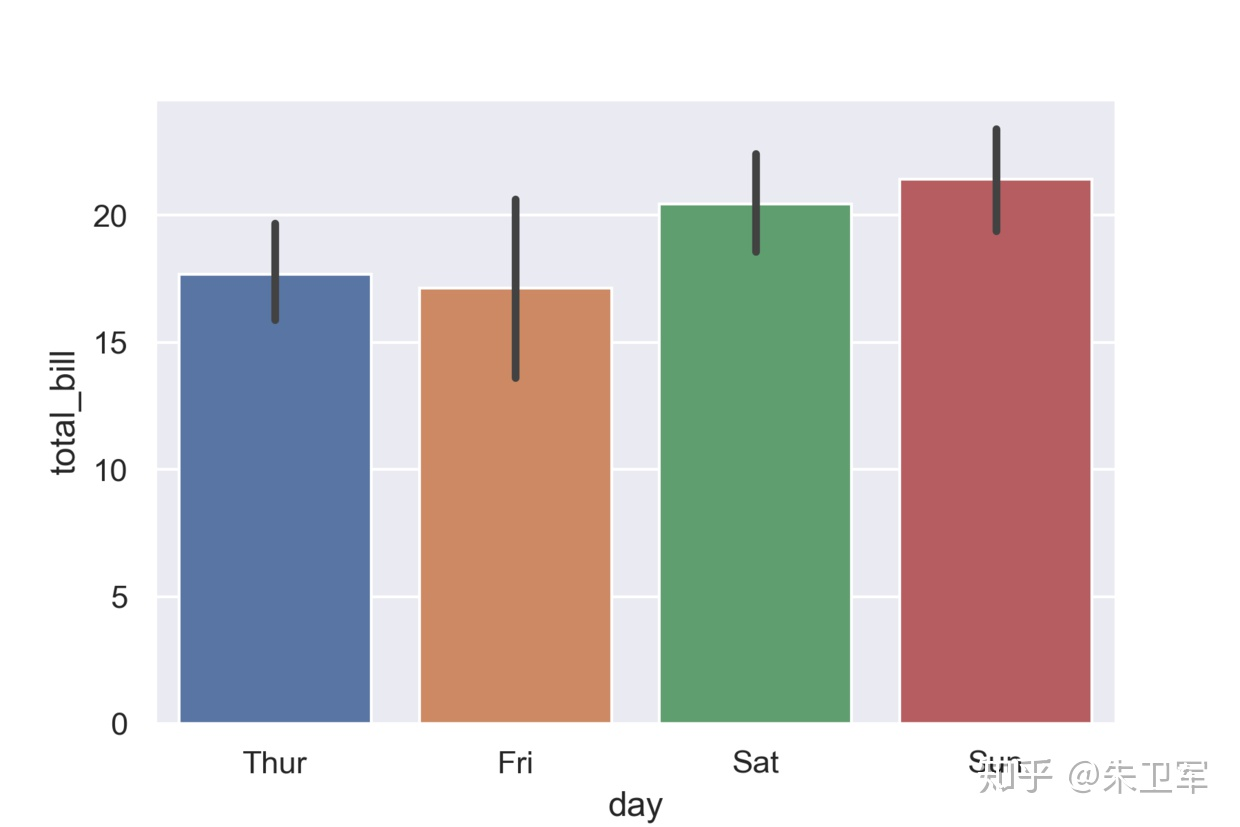3、线型图 函数sns.lineplot绘制折线图和置信区间import seaborn as sns
sns.set()
import matplotlib.pyplot as plt
%matplotlib inline
ax = sns.lineplot(x="timepoint", y="signal", data=fmri)
plt.show()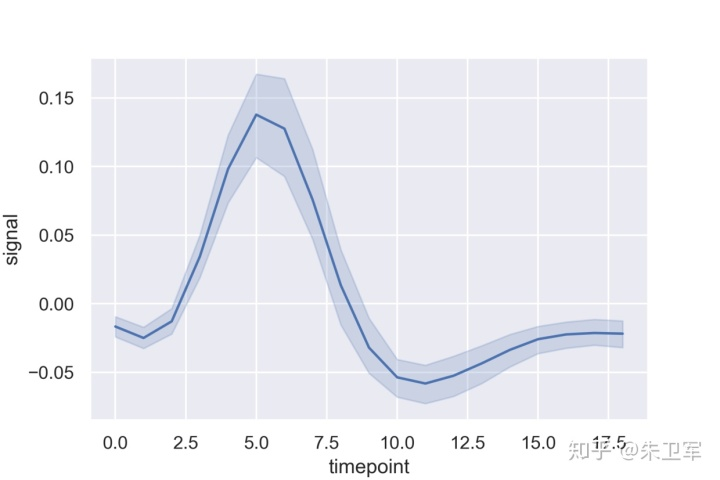4、箱线图 函数seaborn.boxplotimport seaborn as sns
sns.set()
import matplotlib.pyplot as plt
%matplotlib inline
ax = sns.boxplot(x="day", y="total_bill", data=tips)
plt.show()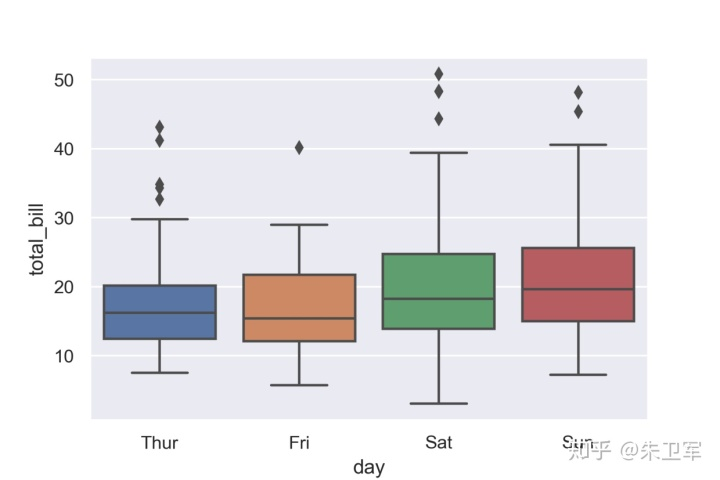5、直方图 函数seaborn.distplotimport seaborn as sns
import numpy as np
sns.set()
import matplotlib.pyplot as plt
%matplotlib inline
np.random.seed(0)
x = np.random.randn(1000)
ax = sns.distplot(x)
plt.show()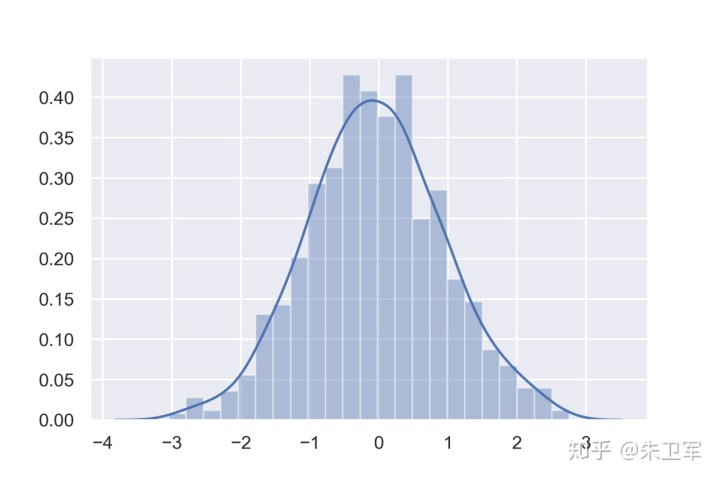6、热力图 函数seaborn.heatmapimport numpy as np
np.random.seed(0)
import seaborn as sns
sns.set()
import matplotlib.pyplot as plt
%matplotlib inline
uniform_data = np.random.rand(10, 12)
ax = sns.heatmap(uniform_data)
plt.show()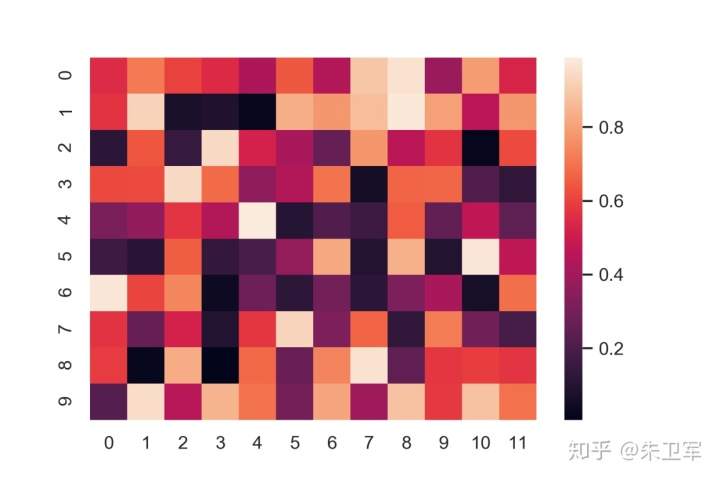7、散点图矩阵 函数sns.pairplotimport seaborn as sns
sns.set()
import matplotlib.pyplot as plt
%matplotlib inline
ax = sns.pairplot(iris)
plt.show()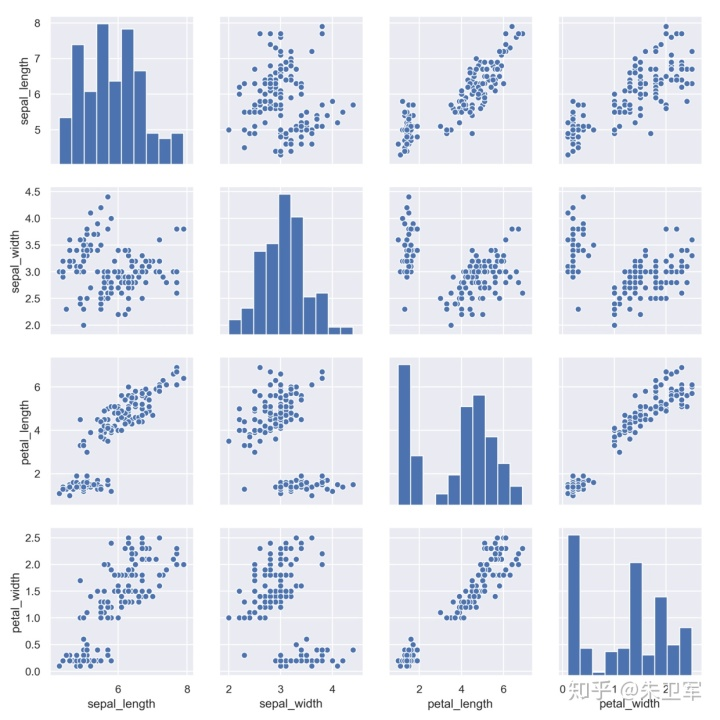8、分类散点图 函数seaborn.catplotimport seaborn as sns
sns.set()
import matplotlib.pyplot as plt
%matplotlib inline
ax = sns.catplot(x="time", y="pulse", hue="kind", data=exercise)
plt.show()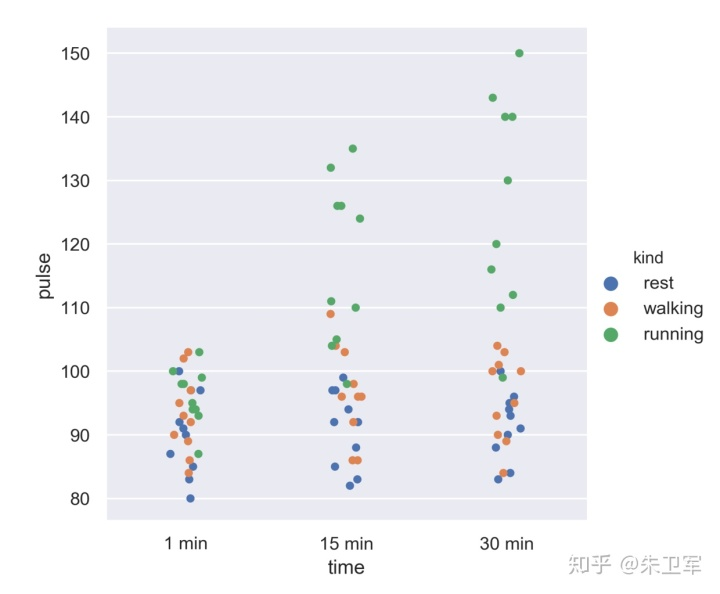9、计数条形图 函数seaborn.countplotimport seaborn as sns
sns.set()
import matplotlib.pyplot as plt
%matplotlib inline
ax = sns.countplot(x="class", data=titanic)
plt.show()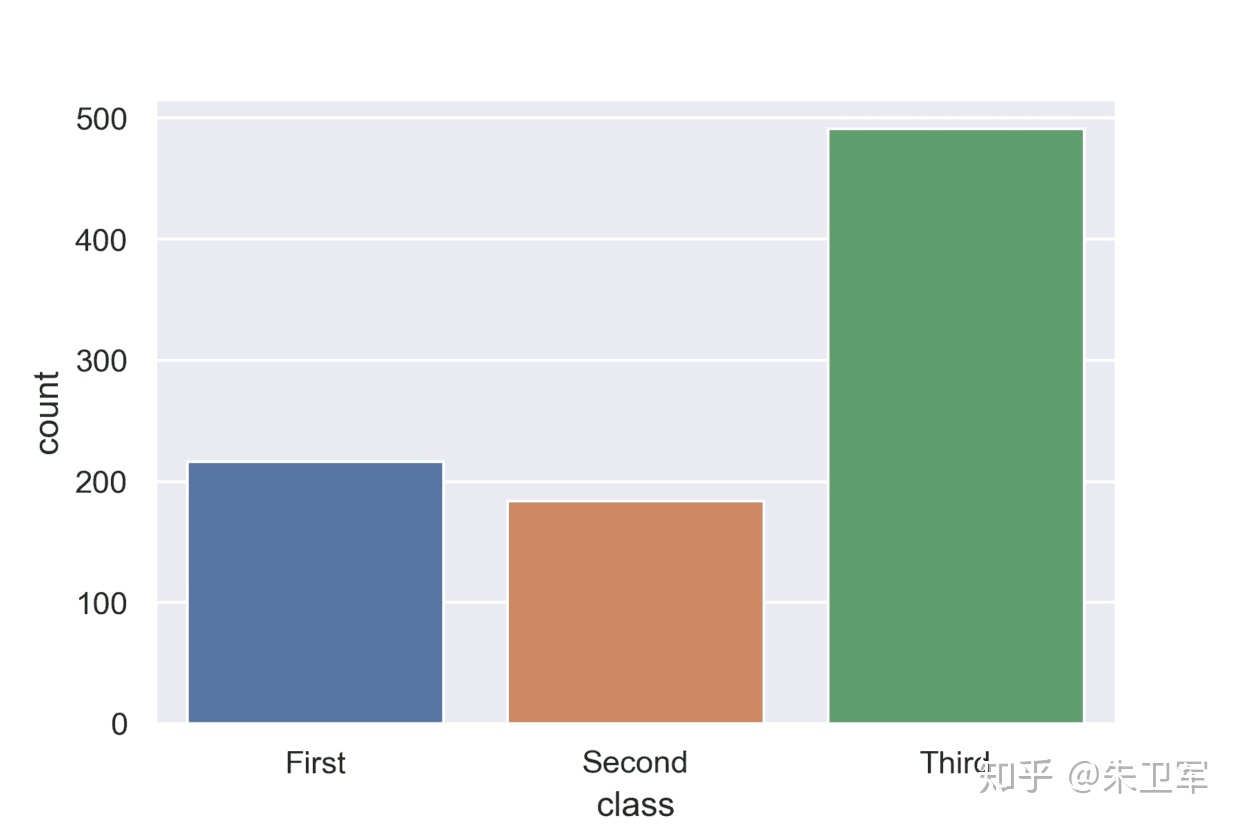10、回归图 函数 seaborn.lmplot绘制散点及回归图import seaborn as sns
sns.set()
import matplotlib.pyplot as plt
%matplotlib inline
ax = sns.lmplot(x="total_bill", y="tip", data=tips)

plt.show()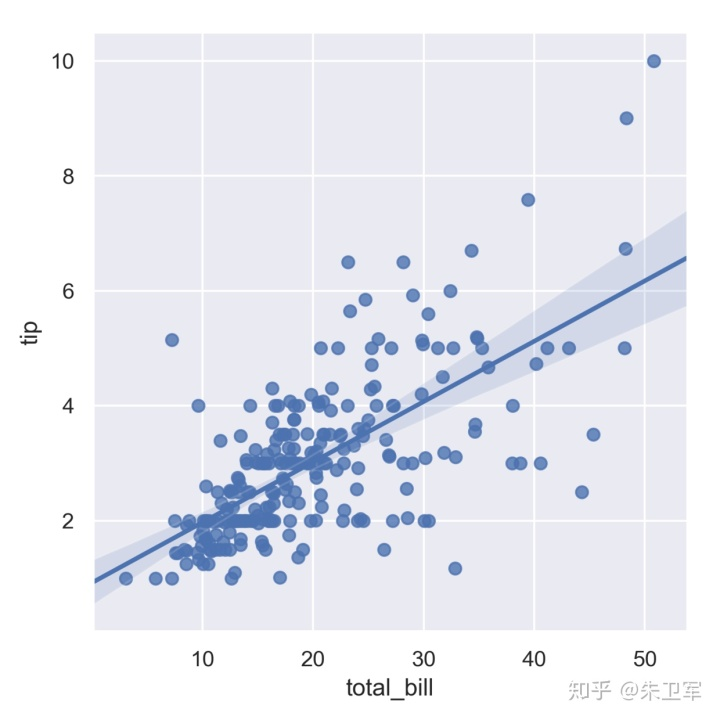展开全文titanic数据集内置示例数据集seaborn内置了十几个示例数据集，通过load_dataset函数可以调用。其中包括常见的泰坦尼克、鸢尾花等经典数据集。# 查看数据集种类import seaborn as snssns.get_dataset_names()import seaborn as sns# 导出鸢尾花数据集data = sns.load_dataset('iris')data.head()1、散点图函数sns.scatterplotimport seaborn as snssns.set()import matplotlib.pyplot as plt%matplotlib inline# 小费数据集tips = sns.load_dataset('tips')ax = sns.scatterplot(x='total_bill',y='tip',data=tips)plt.show()2、条形图函数sns.barplot显示数据平均值和置信区间import seaborn as snssns.set()import matplotlib.pyplot as plt%matplotlib inline# 小费数据集tips = sns.load_dataset("tips")ax = sns.barplot(x="day", y="total_bill", data=tips)plt.show()3、线型图函数sns.lineplot绘制折线图和置信区间import seaborn as snssns.set()import matplotlib.pyplot as plt%matplotlib inlinefmri = sns.load_dataset("fmri")ax = sns.lineplot(x="timepoint", y="signal", data=fmri)plt.show()4、箱线图函数seaborn.boxplotimport seaborn as snssns.set()import matplotlib.pyplot as plt%matplotlib inlinetips = sns.load_dataset("tips")ax = sns.boxplot(x="day", y="total_bill", data=tips)plt.show()5、直方图函数seaborn.distplotimport seaborn as snsimport numpy as npsns.set()import matplotlib.pyplot as plt%matplotlib inlinenp.random.seed(0)x = np.random.randn(1000)ax = sns.distplot(x)plt.show()6、热力图函数seaborn.heatmapimport numpy as npnp.random.seed(0)import seaborn as sns sns.set()import matplotlib.pyplot as plt%matplotlib inlineuniform_data = np.random.rand(10, 12)ax = sns.heatmap(uniform_data)plt.show()7、散点图矩阵函数sns.pairplotimport seaborn as snssns.set()import matplotlib.pyplot as plt%matplotlib inlineiris = sns.load_dataset("iris")ax = sns.pairplot(iris)plt.show()8、分类散点图函数seaborn.catplotimport seaborn as snssns.set()import matplotlib.pyplot as plt%matplotlib inlineexercise = sns.load_dataset("exercise")ax = sns.catplot(x="time", y="pulse", hue="kind", data=exercise)plt.show()9、计数条形图函数seaborn.countplotimport seaborn as snssns.set()import matplotlib.pyplot as plt%matplotlib inlinetitanic = sns.load_dataset("titanic")ax = sns.countplot(x="class", data=titanic)plt.show()10、回归图函数 seaborn.lmplot绘制散点及回归图import seaborn as snssns.set()import matplotlib.pyplot as plt%matplotlib inlinetips = sns.load_dataset("tips")ax = sns.lmplot(x="total_bill", y="tip", data=tips)plt.show()展开全文• 对于初学者来说，很容易被这官网上众多的图表类型给吓着了，由于种类太多，几种图表的绘制方法很有可能会混淆起来。 因此，在这里，我特地总结了六种常见的基本图表类型，你可以通过对比学习，打下坚实的基础。 1. ...
• 欢迎点击右上角关注小编，除了分享技术文章之外还有很多福利...对于初学者来说，很容易被这官网上众多的图表类型给吓着了，由于种类太多，几种图表的绘制方法很有可能会混淆起来。因此，在这里，我特地总结了六种常...python画三维图
• 欢迎点击右上角关注小编，除了分享技术文章之外还有很多福利...对于初学者来说，很容易被这官网上众多的图表类型给吓着了，由于种类太多，几种图表的绘制方法很有可能会混淆起来。因此，在这里，我特地总结了六种常...绘制三维散点图
• 欢迎点击右上角关注小编，除了分享技术文章之外还有很多福利，私信学习资料可以领取包括不限于Python实战演练、PDF电子文档...对于初学者来说，很容易被这官网上众多的图表类型给吓着了，由于种类太多，几种图表的绘...
• 运行时用来部署BIRT，可与java程序或web服务器在API级别或报表查看器级别进行整合 常见报表种类： 表格，图表， 交叉表（按二维方式显示数据）， 信函和文档（方便地生成通知、表格式信函） 创建报表基本步骤 报表...javascript 框架 java web服务
• Matplotlib1. 定义2. 学习目的3. 运用1. 绘制流程2. 三层结构4. 基础绘图功能1. help介绍2. 设置画布属性与图片保存3. 添加x，y轴刻度4.... 折线图应用场景6. 常见图形绘制1....专门用于开发2D图表包括3D图表...可视化 数据可视化 数据分析 人工智能
• FAQ（常见问题解答）、 BBS、聊天室等各种即时信息服务，在线收听、收视、订购、付款等选择性服务，无假日服务和信息跟踪、信息定制到智能化信息转移，网上选购，送货到家上门服务等等，将极大提高顾客满意度...文档
• 鉴于市场上用户手机型号、种类、屏幕分辨率等参差不齐，传统方式根据主流系统分别开发相应系统耗时又耗力，为了高效开发并节约开发项目成本，本文采用Android+HTML5相结合方式进行移动端Web系统设计研发工作...
• 多个数据进行对比这是非常常见的需求，Highstock 可以让你为每个类型的数据添加坐标轴，每个轴可以定义放置的位置，所有的设置都可以独立生效，包括旋转、样式设计和定位，当然也支持多个数据共用一个坐标轴。...
• 包括客户、供应商、产品物料信息编辑、增加、删除等。 7、提醒功能。相关合同审批、待审批提醒、收付款提醒等。 8、搜索查询。可根据条件设定，直接查询合同明细。 9、图表统计分析。提供销售、采购合同图表统计...
• 多个数据进行对比这是非常常见的需求，Highstock 可以让你为每个类型的数据添加坐标轴，每个轴可以定义放置的位置，所有的设置都可以独立生效，包括旋转、样式设计和定位，当然也支持多个数据共用一个坐标轴。...
• 第2篇为实例篇，重点介绍如何使用Excel解决文秘工作中的常见问题，具体包括文秘常用单据制作、办公室常用表格制作、企划书制作、公司会议安排与会议室使用管理、公司车辆使用管理、客户信息管理、员工资料库与...
• 10.1 生成基于DefaultCategoryDataset数据集的图表 实例273 生成水平直方图 实例274 生成水平堆栈图 实例275 绘制3D垂直直方图 实例276 生成垂直堆栈图 实例277 生成区域图 10.2 绘制饼状图表 实例278 生成普通饼图 ...Java
• 10.1 生成基于DefaultCategoryDataset数据集的图表 实例273 生成水平直方图 实例274 生成水平堆栈图 实例275 绘制3D垂直直方图 实例276 生成垂直堆栈图 实例277 生成区域图 10.2 绘制饼状图表 实例278 生成普通饼图 ...Java
• 10.1 生成基于DefaultCategoryDataset数据集的图表 实例273 生成水平直方图 实例274 生成水平堆栈图 实例275 绘制3D垂直直方图 实例276 生成垂直堆栈图 实例277 生成区域图 10.2 绘制饼状图表 实例278 生成普通饼图 ...Java
• 在测试过程中，可能会出现以下常见的几种测试情况： 　一、当事务响应时间的曲线开始由缓慢上升，然后处于平衡，最后慢慢下降这种情形表明： 　* 从事务响应时间曲线图持续上升表明系统的处理能力在下降，事务的响应...
• 用户界面采用视图-对象-状态-操作-权限绑定智能动态工作图表技术，保证了操作简明直观、流畅易用、各司其职；所有录入界面、报表格式、工作视图、功能菜单均支持用户自定义，保证了系统有最大限度自适应性，...
• ## 新版Android开发教程.rar

千次下载 热门讨论 2010-12-14 15:49:11
----------------------------------- Android 编程基础 1 封面----------------------------------- Android 编程基础 2 开放手机联盟 --Open --Open --Open --Open Handset ...• 多媒体支持 包括常见的音频、视频和...
• 提供精美炫酷大屏地图模块，包括静态图片+闪烁效果+迁徙效果+世界地图+区域地图等，可指定点经纬度坐标，识别单击响应，可以做地图跳转等，每个点都可以不同颜色和提示信息，功能超牛逼！ 除了提供大屏系统外...
• 31.启动Windows98中文版后，下列中___C___的图标不是常见的图标。 A、我的电脑 B、回收站 C、资源管理器 D、收件箱 32.多媒体电脑是指 __B___ 。 A、专供家庭娱乐用的电脑 B、能处理文字、图形、影像与声音等信息的...